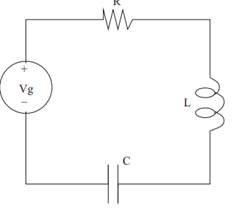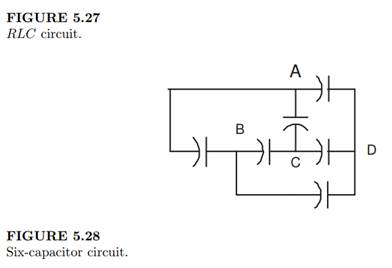### Create an Account

Home / Questions / 1 Find the differential equation for the current in the circuit shown in Figure 527 2 Bet...

# 1 Find the differential equation for the current in the circuit shown in Figure 527 2 Between what pair of points

1. Find the differential equation for the current in the circuit shown in Figure 5.27.

2. Between what pair of points (A, B, C, D) shown in Figure 5.28 should one link up the power supply to charge all six capacitors to an equal capacitance?Jul 26 2020 View more View LessSubscribe To Get Solution